The commands available in this menu change depending upon whether a table, a matrix or a plot is selected.

## Commands for the analysis of data in tables

### Sort Column

The commands in this menu can be used to sort the selected column(s).

#### Ascending

The Analysis -> Sort Column -> Ascending command sorts the selected column or columns in ascending order. If the selection contains more than one column they are sorted independently.

#### Descending

The Analysis -> Sort Column -> Descending command sorts the selected column or columns in descending order. If the selection contains more than one column they are sorted independently.

#### Custom...

The Analysis -> Sort Column -> Custom... command becomes available if there are more than one column selected. This command opens the Sorting Options dialog. In this dialog you have the option to sort the selected columns:

• separately: each column will be sorted independently in ascending or descending order

• together: the column selected as the leading column will be sorted into ascending or descending order. The other selected columns will be sorted so as to keep the rows unchanged.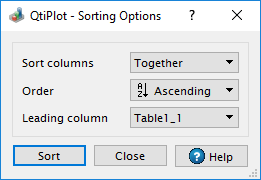### Sort Table

The Analysis -> Sort Table command opens the Sorting Options dialog. It functions in the the same manner as the Custom... command, except that it operates on all columns of the active table.

### Normalize

These commands are used to normalize data in tables. In this case, normalization is the process of dividing each entry in the column by the column's maximum positive value, making the maximum range value equal to 1. Data is not re-centered so negative values will remain negative and the minimum value may be less than -1. Columns are normalized separately. The command does not create new columns for the normalized data but replaces the values in the selected columns with their normalized values. There are 2 variants of this command:

#### Normalize -> Columns

The Analysis -> Normalize -> Normalize -> Columns command normalizes only the selected column or columns. If you want the normalized data in a new column, use the Add Column command to create a new column, fill it using a "copy/paste" sequence and then normalize the new column.

#### Normalize -> Table

The Analysis -> Normalize -> Normalize -> Table command normalizes all the columns of the table. It is not a global normalization of all values of the table: each column is normalized separately.

### Differentiate

The Analysis -> Differentiate command computes the derivative of the selected Y columns using centered finite differences. A new plot is created displaying the result of the numerical differentiation. This command creates a new (hidden) table that contains one column of X-values and one column of derivatives of the Y-values.

### Integrate...

The Analysis -> Integrate... command computes the integral of the selected Y column using the trapezoidal rule. This command opens the Integration Options dialog that allows to specify the data range to be integrated.

### FFT...

The Analysis -> FFT... command computes a direct or inverse Fast Fourier Transform. The parameters used can be set with the FFT dialog. See the fft section of the Analysis chapter for more details.

### Correlate

The Analysis -> Correlate command computes the cross-correlation of the two selected columns. See the correlate section of the Analysis chapter for more details.

### Autocorrelate

The Analysis -> Autocorrelate command computes the cross-correlation of the selected column with itself (auto-correlation). See the correlate section of the Analysis chapter for more details.

### Convolute

Does a convolution of two selected columns. The first one being the response and the second the signal. See the convolution section of the Analysis chapter for more details.

### Deconvolute

The Analysis -> Deconvolute command computes the deconvolution of two selected columns. The first one being the response and the second the signal. See the deconvolution section of the Analysis chapter for more details.

### Analysis -> Fitting

The Analysis -> Fitting menu provides the following data fitting routines for tables:

#### Fit Slope

The Analysis -> Fitting -> Fit Slope command performs a linear fit of the selected curve, using the f(x) = A*x function and the default data fit settings.

#### Fit Linear (Default)

The Analysis -> Fitting -> Fit Linear (Default) command performs a linear fit of the selected curve, using the default data fit settings. The results will be given in the Log panel.

#### Multiple Linear Regression...

The Analysis -> Fitting -> Multiple Linear Regression... command opens the Multiple Linear Regression dialog.

#### Fit Wizard... (Ctrl-Y)

Opens the Fit Wizard dialog, allowing you to choose the curve to fit, the algorithm and tolerance to use, and the number of iterations to be performed. You also enter the analytical function, the names of the fitting parameters and their initial (guessed) values. See the Data Fitting section of the Analysis chapter for more details.

## Commands for the analysis of curves in plots

The following items are enabled only if the active window is a 2D Multilayer Plot Window. If the active plot layer contains more than one curve, and the Data Range Selectors are not enabled, a dialog window will pop-up allowing you to select the curve you want to analyze.

In most cases (except for integration), a new red curve is added to the active plot layer and a new table containing the data used to plot this curve is added to the workspace. Useful information about the operation performed will be shown in the Results Log display.

The commands for theFFT... and Fit Wizard... are presented in the Table Analysis Menu.

### Translate

This next group of are 2 commands are used for translating curves horizontally and vertically.

#### Vertical

The Analysis -> Translate -> Vertical command is used to move a curve in the vertical direction. When the command is selected, the cursor changes to a box and cross with an X-Y coordinate display which floats along with the cursor. You then select 2 points. The first must be a point on a curve. When the first points is selected, a crosshair will be drawn to indicate it's location. The second point can then be selected anywhere in the active graph region. The vertical distance between the selected points will be calculated, and the selected curve will be translated by this amount. The net result is to place the selected graph point at the new vertical location. Horizontal distance is ignored. This allows selection of the second point at one of the vertical axes, facilitating alignment of the selected graph point with some value on that axis. The second point may also be another curve point (either on the same or a different curve), a feature which makes it easy to align two or more curves in the vertical direction. Warning: the underlying curve's data is modified by this operation. No other warning will be given.

#### Horizontal

The Analysis -> Translate -> Horizontal command is used to move a curve in the horizontal direction. When the command is selected, the cursor changes to a box and cross with an X-Y coordinate display which floats along with the cursor. You then select 2 points. The first must be a point on a curve. When the first points is selected, a crosshair will be drawn to indicate it's location. The second point then can be selected anywhere on the active graph region. The horizontal distance between the selected points will be calculated, and the curve will be translated horizontally by this amount. The net result is to place the selected graph point at the new location. Vertical distance is ignored. This allows selection of the second point at one of the horizontal axes, facilitating alignment of the selected graph point with some value on that axis. The second point may also be another curve point (either on the same or a different curve), a feature which makes it easy to align two or more curves in the horizontal direction. Warning: the underlying curve's data is modified by this operation. No other warning will be given.

### Subtract

The Subtract group is also used to translate curves, however, in these cases, some functional relationship is used.

#### Subtract -> Baseline

The Analysis Subtract -> Baseline command opens the Baseline dialog.

#### Subtract -> Reference Data

The Analysis Subtract -> Reference Data command opens the Math on Data Sets dialog which makes possible to perform arithmetic operations on data curves.

#### Subtract -> Straight Line

The Analysis Subtract -> Straight Line command operates in a manner similar to the Analysis -> Subtract -> Baseline command. However, in this case, the two points which are selected define the end-points of a straight line which is subtracted from all the curves on the layer.The cursor changes to a cross target with an X-Y coordinate display which floats along with the cursor, similar to that used in the Analysis -> Subtract -> Baseline command. When the first point is selected, it will be marked with a small circle and crosshairs. As soon as the second point is selected, the line between the two points will be subtracted from the curves. Warning: the underlying curves' data is modified by this operation. No other warning will be given.

### Peaks...

The Analysis -> Peaks... command opens the Find Peaks dialog.

### Differentiate

The Analysis -> Differentiate command creates a new plot displaying the resulting curve of the numerical differentiation. The computation of the derivative is done by centered finite differences. A new table is created which contains one column for X-values and one column for derivatives of Y-values. It also creates a new plot of the derivative.

### Integrate...

The Analysis -> Integrate... command performs a piecewise numerical integration of a curve on the selected layer. Curves are integrated using the trapezoidal rule. There must be at least one curve on the plot layer. This command opens the Integration Options dialog that allows to specify the data range to be integrated.

### Integrate Function...

The Analysis -> Integrate Function... command provides the capability of performing the numerical integration of a user defined function. The Integrate Function dialog is opened when this command is executed. This dialog provides for the definition of the function, the variable of integration, and the parameters that control the integration. Integration is performed using the QAGS algorithm from the GNU Scientific Library. For many functions, all you need do is set the limits of integration and click the "Integrate" button. The default values for the other parameters will usually produce excellent results. For some functions, especially those containing singularities and discontinuities, you may need to experiment with the number of sub-intervals and the tolerance limit to obtain acceptable results. However, a detailed discussion of the use of these 2 parameters is beyond the scope of this manual. If the Plot Area option is checked, a curve of the integrated function will be added to the currently active layer. In any case, an entry is made in the Log Panel which contains the numerical result of the computed definite integral over the specified interval.

### Smooth

There are 4 variants of the Smooth menu item:

#### Savitski-Golay...

The Analysis -> Smooth -> Savitsky-Golay... command performs a smoothing of the selected curve using the Savitzky-Golay method. The formula used to smooth the curve defined by the points yi=f(xi) is:The fi values are computed by fitting the data points to a polynomial. They depend on the number of points used for the smoothing of the curve and the order of the polynomial. Compared to the moving window average method, the advantage of this smoothing method is that the values of extrema are not truncated. The dialog allows specification of the curve which will be smoothed, the order of the polynomial, the number of data points used for the polynomial fit before and after each point, and the color used to draw the smoothed curved. A new table will be created to store the data points xi, zi.

Figure 3-1. The Smooth -> Savitsky-Golay... dialog.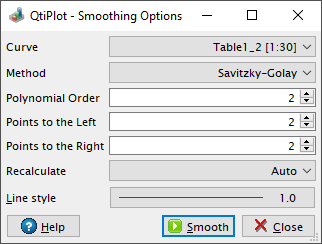#### Moving Window Average...

The Analysis -> Smooth -> Moving Window Average... command performs a smoothing of the selected curve with the moving window average method. The formula used to smooth the curve defined by the points yi=f(xi) is: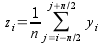The greater the number of points, n, the smoother the resulting curve zi=f(xi) will be. The dialog allows specification of the curve which will be smoothed, the value of n and the color used to draw the smoothed curve. A new table will be created to store the data points xi, zi.

Figure 3-2. The Smooth -> Moving Window Average... dialog.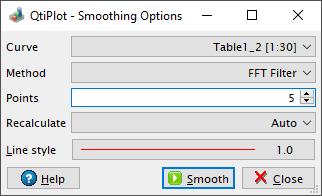#### Lowess...

The Analysis -> Smooth -> Lowess... command performs a smoothing of the selected curve using the Lowess (aka Loess) algorithm. It provides a robust locally weighted regression and is well suited to smooth data for which no formal model exists.

The parameter f is the fraction of points which define the local neighborhood. A value of 0.2 uses 20% of the curve total points as neighbors for each data point (+/- 10%). Values of f closer to 1 yield smoother curves. The iterations parameter specifies the number of times to run the algorithm runs over the entire data set, each time refining the local weights. In most cases, two iterations will be sufficient.

Figure 3-3. The Smooth -> Lowess... dialog.#### FFT Filter...

The Analysis -> Smooth -> FFT Filter... command performs a smoothing of the selected curve using the Low Pass method. The number of points specified is used to calculate a cut-off frequency. The dialog also allows specification of the curve which will be smoothed and the color used to draw the smoothed curve. A new table will be created to store the data points of the smoothed curve.

Figure 3-4. The Smooth -> FFT Filter... dialog.### FFT Filter

The Analysis -> FFT Filter menu item provides Lowpass, Highpass, Bandpass and Bandreject filter topologies:

#### Low Pass...

The Analysis -> FFT Filter -> Low Pass... command uses an FFT based digital filter to attenuate the high frequencies present in an input signal. See the filtering section for more details. This command opens the FFT Filter Dialog with the filter type set to Low Pass FFT Filter. In this dialog you can select the curve (input signal) to filter and the cut-off frequency of the filter.

Figure 3-5. The FFT Filter -> Low Pass... dialog.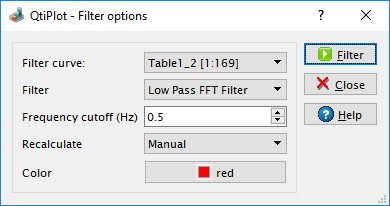This command creates a new table containing the filtered data, and adds a new curve to the current layer. The curve is a plot of the filtered data.

#### High Pass...

The Analysis -> FFT Filter -> High Pass... command uses an FFT based digital filter to attenuate the low frequencies present in an input signal. See the filtering section for more details. This command opens the FFT Filter Dialog with the filter type set to High Pass FFT Filter. In this dialog you can select the curve to filter and the cut-off frequency of the filter.

Figure 3-6. The FFT Filter -> High Pass... dialog.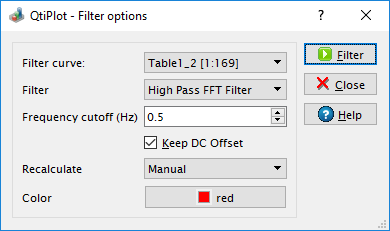This command creates a new table containing the filtered data, and adds a new curve to the current layer. The curve is a plot of the filtered data.

#### Band Pass...

The Analysis -> FFT Filter -> Band Pass... command uses an FFT based digital filter to attenuate both high and low frequencies present in an input signal. See the filtering section for more details. This command opens the FFT Filter Dialog with the filter type set to Band Pass FFT Filter. In this dialog you can select the curve to filter and both the low and high cutoff frequencies of the filter.

Figure 3-7. The FFT Filter -> Band Pass... dialog.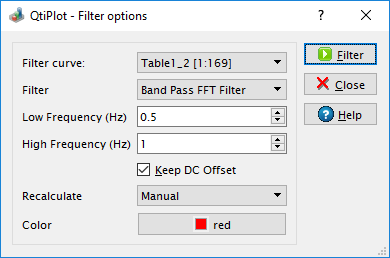This command creates a new table containing the filtered data, and adds a new curve to the current layer. The curve is a plot of the filtered data.

#### Band Block...

The Analysis -> FFT Filter -> Band Pass... command uses an FFT based digital filter to remove a band of frequencies from a signal while leaving those frequencies above and below the stop band. See the filtering section for more details. This command opens the FFT Filter Dialog with the filter type set to Band Block FFT Filter. In this dialog you can select the curve to filter and both the lower and upper stop-band frequencies of the filter.

Figure 3-8. The FFT Filter -> Band Block... dialog.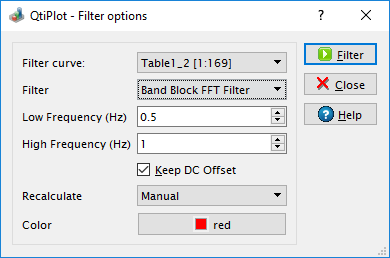This command creates a new table containing the filtered data, and adds a new curve to the current layer. The curve is a plot of the filtered data.

#### Math on Data Sets...

The Analysis -> Math on Data Sets... command opens the Math on Data Sets dialog which makes possible to perform arithmetic operations on data curves.

#### Average Multiple Curves...

The Analysis -> Average Multiple Curves... command opens the Average Multiple Curves dialog which makes possible to average multiple input curves in order to create a new one.

### Interpolate...

The Analysis -> Interpolate... command performs an interpolation using the selected method. The curve must have enough data points to compute interpolated points, if not a warning message will be popped up.

The available interpolation methods are Linear (the curve must contain at least 3 points), Cubic Spline (the curve you analyze must contain at least 4 points), Non-rounded Akime spline (the curve you analyze must contain at least 5 points). See the Analysis chapter for a comparison of the different methods.

Figure 3-9. The Interpolate... dialog.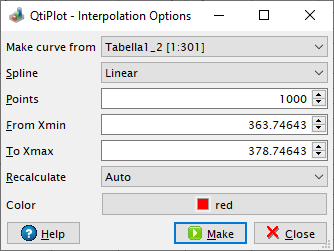This command creates a new curve on the current layer, and a new table.

### FFT...

The Analysis -> FFT... command performs a forward or inverse FFT of the selected curve. The parameters used can be set with the FFT dialog.

The inverse FFT transform of a forward transform will result in a data set identical to that used for the forward transform.

### Analysis -> Fitting

The Analysis -> Fitting menu provides the following data fitting routines for plot curves:

#### Fit Slope

The Analysis -> Fitting -> Fit Slope command performs a linear fit of the selected curve, using the f(x) = A*x function and the default data fit settings.

#### Fit Linear (Default)

The Analysis -> Fitting -> Fit Linear (Default) command performs a linear fit of the selected curve, using the default data fit settings. The results will be given in the Log panel.

#### Fit Linear...

The Analysis -> Fitting -> Fit Linear... command opens the Linear Fit Options dialog, allowing to choose the curve to fit, the number of points for the resulting curve and the abscissa limits for the fit as well as some other data fit options.

#### Fit Polynomial...

The Analysis -> Fitting -> Fit Polynomial... command opens the Polynomial Fit Options dialog, allowing to choose the curve to fit, the order of the polynomial function to use, the number of points of the resulting curve and the abscissa limits for the fit.

#### Fit Exponential Decay

Fit Exponential Decay provides 3 forms of exponential decay:

##### First Order...

The Fit Exponential Decay -> First Order... opens the Fit Exponential Decay dialog, allowing you to choose the curve to fit and the initial guesses for the fit parameters.

##### Second Order...

The Fit Exponential Decay -> Second Order... opens the Fit Exponential Decay - Second Order dialog, allowing you to choose the curve to fit and the initial guesses for the fit parameters.

##### Third Order...

The Fit Exponential Decay -> Third Order... opens the Fit Exponential Decay - Third Order dialog, allowing you to choose the curve to fit and the initial guesses for the fit parameters.

#### Fit Exponential Growth...

Analysis -> Fitting -> Fit Exponential Growth... opens the Fit Exponential Growth Dialog, allowing to choose the curve to fit and the initial guesses for the fit parameters.

#### Fit Boltzmann (sigmoidal)

The Analysis -> Fitting -> Fit Boltzmann (sigmoidal) performs a fit to a Boltzmann function on the selected curve. It can be used to obtain the correlation equation of an S shaped data set.

#### Fit Pseudo-Voigt

Analysis -> Fitting -> Fit Pseudo-Voigt provides two forms of Pseudo-Voigt profile:

##### PsdVoigt1...

The Fit Pseudo-Voigt -> PsdVoigt1... performs a fit to a PsdVoigt1 function on the selected curve.

##### PsdVoigt2...

The Fit Pseudo-Voigt -> PsdVoigt2... performs a fit to a PsdVoigt2 function on the selected curve.

#### Fit Gaussian

The Analysis -> Fitting -> Fit Gaussian performs a Gaussian fit to the selected curve. It can be used to obtain the correlation equation of a bell shaped data set.

#### Fit Lorentzian

Analysis -> Fitting -> Fit Lorentzian performs a Lorentzian fit to the selected curve. It can be used to obtain the correlation equation of a bell shaped data set.

#### Fit Multi-peak

Fit Multi-peak performs a fit to a sum of profile functions on the selected curve. Four different profiles are available:

##### Gaussian...

Fit Multi-peak -> Gaussian... performs a fit to a sum of N Gaussian functions on the selected curve.

##### Lorentzian...

Fit Multi-peak -> Lorentzian... performs a fit to a sum of N Lorentzian functions on the selected curve.

##### PsdVoigt1...

Fit Multi-peak -> PsdVoigt1... performs a fit to a sum of N Pseudo-Voigt functions on the selected curve.

##### PsdVoigt2...

Fit Multi-peak -> PsdVoigt2... performs a fit to a sum of N Pseudo-Voigt functions on the selected curve.

#### Fit Wizard... (Ctrl-Y)

Opens the Fit Wizard dialog, allowing you to choose the curve to fit, the algorithm and tolerance to use, and the number of iterations to be performed. You also enter the analytical function, the names of the fitting parameters and their initial (guessed) values. See the Data Fitting section of the Analysis chapter for more details.

## Commands for the analysis of data in matrices

The following items are enabled only if the active window is a matrix window.

### Integrate

The Analysis -> Integrate command computes the integral of the selected matrix using the trapezoidal rule. The result will be displayed in the Log panel.

### FFT...

The Analysis -> FFT... command computes a direct or inverse two dimensional Fast Fourier Transform. The parameters used can be set with the FFT dialog. See the FFT section of the Analysis chapter for more details.

### Forward FFT

The Analysis -> Forward FFT command computes a direct two dimensional Fast Fourier Transform. Please beware that the input matrix will be modified in order to store the amplitudes of the 2D FFT operation.

### Inverse FFT

The Analysis -> Inverse FFT command computes an inverse two dimensional Fast Fourier Transform. Please beware that the input matrix will be modified in order to store the amplitudes of the 2D FFT operation.

### FFT Filter

The Analysis -> FFT Filter menu item provides Lowpass, Highpass, Bandpass and Bandreject filters. QtiPlot uses the two dimensional FFT method explained in the following article by Paul Bourke: http://paulbourke.net/miscellaneous/dft/.

#### Low Pass...

The Analysis -> FFT Filter -> Low Pass... command uses a two dimensional FFT based digital filter to attenuate the high frequencies present in an input matrix, see the following article by Paul Bourke http://paulbourke.net/miscellaneous/imagefilter/ for more details. This command opens the FFT Filter Dialog with the filter type set to Low Pass FFT Filter. In this dialog you can select the matrix to filter and the cut-off frequency of the filter. The input matrix will be modified in order to store the filtered data.

#### High Pass...

The Analysis -> FFT Filter -> High Pass... command uses a 2D FFT based digital filter to attenuate the low frequencies present in an input matrix, see the following article by Paul Bourke http://paulbourke.net/miscellaneous/imagefilter/ for more details. This command opens the FFT Filter Dialog with the filter type set to High Pass FFT Filter. In this dialog you can select the matrix to filter and the cut-off frequency of the filter. The input matrix will be modified in order to store the filtered data.

#### Band Pass...

The Analysis -> FFT Filter -> Band Pass... command uses a 2D FFT based digital filter to attenuate both high and low frequencies present in an input signal, see the following article by Paul Bourke http://paulbourke.net/miscellaneous/imagefilter/ for more details. This command opens the FFT Filter Dialog with the filter type set to Band Pass FFT Filter. In this dialog you can select the matrix to filter and both the low and high cutoff frequencies of the filter. The input matrix will be modified in order to store the filtered data.

#### Band Block...

The Analysis -> FFT Filter -> Band Pass... command uses a 2D FFT based digital filter to remove a band of frequencies from a matrix while leaving those frequencies above and below the stop band, see the following article by Paul Bourke http://paulbourke.net/miscellaneous/imagefilter/ for more details. This command opens the FFT Filter Dialog with the filter type set to Band Block FFT Filter. In this dialog you can select the matrix to filter and both the lower and upper stop-band frequencies of the filter. The input matrix will be modified in order to store the filtered data.